The Manifesto | The Dominion | Texts and Articles | Review | Links | ACCUEIL (FR) | HOME (EN)

 Planetary Aspects and Terrestrial Earthquakes by Brian T. Johnston

Astrologers since ancient times have always asserted that the planets influence earthquakes. The timing of earthquakes has largely been determined by examining the timing of planetary aspects. That is, when the planets form precise geometric angles to one another earthquakes are supposed to occur. While the astrological community has generally accepted this theory the scientific establishment has largely ignored and ridiculed such assumptions. For the astronomers this would seem to imply effect at a distance, something that they have ruled out as possible. During the course of many years of astrological research it was noted that earthquakes did indeed seem to be associated with the aspects and many significant earthquakes seemed to be associated with major planetary aspects. Another astrological premise is that applying aspects, that is aspects between the planets that have not yet fully formed their perfect angles, are more active than separating aspects, i.e., ones that have already passed the exact angle. As an example the magnitude 5.7 earthquake that occurred in Jawa, Indonesia on March 12, 2001 at 23:35:08 UT, at 7S02 and 106E05 had aspects of Sun semi-sextile Uranus seven minutes applying, Sun semi-square Neptune, twenty-four minutes applying and Mercury square Saturn 58 minutes applying. Thus, this earthquake would be considered to be under an applying aspect as the Sun was applying by semi-sextile to Uranus at an angle of 30° 07.

Astrological theory states that the change in the level of activity initiated by the applying aspects should be apparent as an increase in the level of geoseismicity. The number of earthquakes that occur during applying aspects should be significantly higher during periods of applying aspects when compared to periods of separating aspects.

Random earthquakes were observed over a period spanning many years in order to see if there was a correlation between the angular positions of the planets and the occurrence of quakes on the earth. What was found was that there appeared to be more earthquakes when there was an abundance of planetary aspects. From this beginning there began a program to try to predict when earthquakes would occur. The results were not very promising, but there was enough correlation to proceed with further investigations. An analysis of a series of randomly selected great earthquakes that occurred during the twentieth century was undertaken. There did seem to be more exact angular relationships between the planets than was to be expected, but this is a condition that is actually much more difficult to determine than it would at first appear. This difficulty arises due to the fact that the motions of the planets as observed from the earth follow a rather tortuous path and it cannot be easily determined what the probability of any given position of a planet will be. These apparent positions are referred to as the geocentric co-ordinates of the planets. The positions of the planets as viewed from the sun, their heliocentric positions, are much more easily predicted. These, like the geocentric positions, also seemed to indicate an excess of planetary aspects when the earthquakes were occurring, although what exactly was happening during these times was extremely difficult to determine. Attempts at producing control charts to compare against the earthquake charts were initially largely unsuccessful. This was due to the fact that repeating cycles of planetary positions as viewed from the earth and the uneven apparent motions of the planets muddied all the results. It was somewhat easier to propagate a control group for the heliocentric positions of the planets. These comparisons did indicate that there were more aspects occurring during the earthquakes that during the randomly selected times, but the statistical relationships were not strong. One of the problems that arose was that the exact times of the earthquakes that were under study were not known precisely and this factor added too much uncertainty to the results. When the earthquake data collecting began in the 1970s the circumstances surrounding the quakes such as their exact time and location were not easily obtained. This was before the era of the Internet and the posting on it by the U.S. Geological Survey and others of the exact times, positions and magnitudes of earthquakes. The Internet increased accessibility to the relevant data and this has facilitated the study thousands of quakes and has thus eased the attempt to find any correlation between the times that they occur and the corresponding positions of the planets in space.

First obtained was the data for the most significant earthquakes of 1998 as published by the U.S. Geological Survey. This set was comprised of eighty-four events. The astrological program "Solar Fire" was used to calculate the positions of the planets and their apparent positions in the sky at the exact moment and position on the earth to the nearest arc minute of longitude and latitude and to the nearest second in time of the quakes. Next was calculated the exact geocentric positions for the planets at the moment of each quake were calculated. From these times and dates there was generated a control group of charts that were made by using a random number generator to determine the dates for an equal number of random sky charts for 1998. This generated a data base of planetary positions and relationships that would give a very similar configuration of planetary positions as the earthquake data, but would be sufficiently different enough from the earthquake times to provide a completely dissimilar set of planetary configurations.

The initial results from the earthquake and random data bases using the angles of 60, 120, 180, and 0 degrees, the sextile, trine, opposition and conjunction aspects, showed that more aspects were present during the moments that earthquakes occurred than during the random periods. The difference between the two sets of data was not large, but it indicated that the initial presumptions regarding the observations that more aspects were occurring during earthquakes than at other times was supported. There were 781 aspects for the random group and 800 for the earthquake group.

The second set, utilizing the same aspect criteria, was generated from the heliocentric positions of the planets for both random times and the times that earthquakes occurred showed very similar results with 539 aspects occurring during the times that earthquakes occurred and 511 aspects for the random group. This was a slightly greater correlation than for the geocentric group and again supported the theory that more aspects were in force during times of aspects that during random times.

The standard orbs, the span during which the aspect is considered to be in force, were comparatively large and were actually in effect for several days before and after the earthquakes actually happened. To more closely examine the exact period of the actual earthquakes the "orb" was reduced to plus or minus one degree of arc. Two more aspect databases were generated and then compared. The earthquakes had 132 geocentric aspects and the random database produced 125 aspects. This is exactly the same ratio as for the first group of geocentric positions and standard orbs and further supported the theory that aspects were more abundant during periods when earthquakes occurred than during random times. The statistical strength of these ratios was not very pronounced and suggested that the results could be due to random error. To further reduce this possibility another set of events was generated which consisted of the ninety- six most significant earthquakes that happened during 1997 as determined by the USGS. The same type of analysis was carried out with these events as for the earthquakes of 1998. The random set was generated in a different manner, however. The random group was generated by taking the same time and place of the actual earthquake and subtracting three days from that moment and generating a new set of planetary positions for the new time. The results showed that there were again more aspects in force, using the standard orbs, than during the period three days before with 341 aspects in effect during the earthquakes and 307 during the random times. As a result of these findings the relationship between earthquakes and planetary positions appeared to be getting stronger.

In order to determine if applying aspects were more predominant than separating aspects during earthquakes I looked at what aspect had the smallest orb when the earthquake occurred and noted whether, or not it was applying, or separating. For the 1996 group there were 42 earthquakes occurring during an applying aspect and 38 during a separating aspect. During 1997 there were 58 earthquakes with applying aspects and 38 with separating aspects. In 1998 there were 46 earthquakes that occurred when applying aspects dominated, while there were again 38 earthquakes with separating aspects. Once more this reinforced the notion that the aspects were generating higher levels of seismic activity than when compared to times when there were no aspects. Eventually 38 groups of earthquakes were generated from earthquakes that occurred from 1975 to 2000 of about 30 events each. (see Table 1.) Percentages of each group that were applying were compared to randomly generated percentages. These two sets of data were then compared and a t-test was performed upon the results. This t-test illustrated that the probability that the results were due to random errors was very remote. (see T-test 1.) Note: for all t-tests variable 1 is random.

T-test 1 for all data bases examined

 t-Test: Two-Sample Assuming Equal Variances Variable 1 Variable 2 Mean 50.62162 61.81081 Variance 70.46396 117.0465 Observations 37 37 Pooled Variance 93.75526 Hypothesized Mean Difference 0 df 72 t Stat -4.97035 P(T<=t) one-tail 2.18E-06

The most noticeable difference between these two groups of numbers is that the mean for the earthquake group is 61.8% of all earthquakes occurred during applying aspects, while for the random group they accounted for only 50.62% of the whole. We can also look at this study in a different manner to determine the probability that the results are due to random error. The probability that any given aspect is either applying, or separating is 50%. While there is a slight excess of applying aspects over separating aspects due to the idiosyncrasies of retrogradation, this effect is only seen with planets that appear to go through retrograde motion. The bulk of the total planetary aspects are with the sun and the moon, interactions with the planets and that are why there is a 0.62% excess of applying aspects in the random group. For all practical purposes we can assume that the relationship between applying and separating aspects is exactly the same as flipping a coin. The probability of a coin toss resulting in a non-random distribution for heads being up is 1 divided by two to the power of the number of times that you toss the coin. In this case we have only one set of earthquakes with a less than 50% distribution of applying aspects. Therefore, we have 2 divided by two to the 37th power as the probability that the results are due to random error. This is equal to approximately 275 billion to one odds against chance. It seems very unlikely that this would occur when flipping coins. This series however, is very fortuitous due to the fact that that the investigations were specifically looking for positive results. Some of the databases had inadvertently included overlapping data. If we look at all earthquakes and sequential databases that include all aspects the number of positive results reduces to about 70%. This would reduce the random chance in the above series to about 68 million to one odds. A t-test performed on a more critical subset of 30 databases demonstrates that there is a very low probability that the results are due to chance. These databases remove all overlapping data from the larger series that was previously analyzed.

T-test 2 for non-overlapping databases

 t-Test: Two-Sample Assuming Equal Variances Variable 1 Variable 2 Mean 50.3 61.76666667 Variance 77.38965517 120.8057471 Observations 30 30 Hypothesized Mean Difference 0 df 55 t Stat -4.461193158 P(T<=t) one-tail 2.03653E-05 t Critical one-tail 1.673033694

During the next study the various aspects were examined to determine which were affecting the general seismicity of the earth. It was found that the conjunction, semi-square, sextile, square, trine, sesqui-quadrate and opposition were the only aspects that resulted in non-random effects when evaluated. When any other aspects, such as the septile, or novile, were added to the mix, then the results turned out to be random. One interesting thing to note is that when only aspects of the eighth harmonic used the results always tended to increase the number of applying versus separating aspects. For the final study 360 earthquakes were randomly selected and a control group of 360 non-earthquake horoscopes were constructed.

The control group was constructed by taking earthquake times and subtracting three months and three days from the earthquake times and then erecting the resultant horoscopes. For each group the results were analyzed comparing the total number of applying and separating aspects that were dominant in each chart. The determination of the dominant aspect was determined by observing what aspect was closest to the point of perfection and whether, or not that aspect was applying, or separating. An aspect was considered to be any planetary combination involving angles of the twenty fourth harmonic that is traditionally considered to be an aspect. In other words the only "aspects" under consideration are the conjunction (0° ), semi-sextile (30° ), semi-square (45° ), sextile (60° ), square (90° ), trine (120° ), sesqui-quadrate (135° ), inconjunct (150° ) and opposition (180° .) Previous studies showed that any inclusion of other angles from the 24th, or any other harmonic, resulted in an increase in the randomization of the results. The only angles that resulted in a decrease in random results were the angles above. The 360 earthquakes were randomly selected from a random database of earthquakes that included events that occurred over the last approximately 100 years. Most of the events occurred during the 1990s, a large number were from May, 1975 and a small number from 2000. All of the events were courtesy of the United State Geological Surveys earthquake databases available on their web site. All events had the exact time to the nearest second recorded and the position to the nearest minute of arc. The random events were recorded to the same level of accuracy.

The initial results of the trial showed that there were 61.8% of the earthquake charts that had applying aspects as the closest aspect, while the random group had 49% applying aspects and 49% separating aspects and 2% with no aspects less than 1 degree. In other words there were 61.8% of the earthquake charts with applying aspects and 38.2% with separating aspects. The random charts were virtually evenly split between applying and separating charts. Three hundred charts from each database were then divided into groups of 10. These 30 groups of earthquake and random charts were then analyzed by t-tests giving results that were very significant and virtually ruled out any possibility of the results being due to random error. The first t-test shows the probability that differences between the earthquake and random groups are not likely due to random error :

 t-Test 1 : Paired Two Sample for Means Variable 1 Variable 2 Mean 11.6 17.93333 Variance 19.62759 19.23678 Observations 30 30 Pearson Correlation -0.8763 Hypothesized Mean Difference 0 Df 29 t Stat -4.06229 P(T<=t) one-tail 0.000169 t Critical one-tail 1.699127 P(T<=t) two-tail 0.000338 t Critical two-tail 2.045231

If we hypothesize that the mean difference will be 3.3 for each group of thirty events then the results give a
Virtually eliminates the probability that the results are due to chance. (The value of 3.3 is derived from the fact that 61% of 30 is 18.3 and 50% is 15, thus the mean difference should be 3.3.)

 t-Test 2: Paired Two Sample for Means Variable 1 Variable 2 Mean 14.6 17.93333 Variance 10.17931 19.23678 Observations 30 30 Pearson Correlation 0.217343 Hypothesized Mean Difference 3.3 Df 29 t Stat -7.52149 P(T<=t) one-tail 1.36E-08 t Critical one-tail 1.699127 P(T<=t) two-tail 2.73E-08 t Critical two-tail 2.045231

A third t-test was performed to determine if the difference between the number of applying versus separating aspects for the earthquake charts was significant. The results for this test were that the probability that the results were due to chance was very low.

 t-Test 3: Paired Two Sample for Means Variable 1 Variable 2 Mean 11.6 17.93333 Variance 19.62759 19.23678 Observations 30 30 Pearson Correlation -0.8763 Hypothesized Mean Difference 0 df 29 t Stat -4.06229 P(T<=t) one-tail 0.000169 t Critical one-tail 1.699127 P(T<=t) two-tail 0.000338 t Critical two-tail 2.045231

If we assume that the difference between the number of earthquakes with applying aspects will be 6 then the probability against chance dramatically increases.

 t-Test 4: Paired Two Sample for Means Variable 1 Variable 2 Mean 11.6 17.93333 Variance 19.62759 19.23678 Observations 30 30 Pearson Correlation -0.8763 Hypothesized Mean Difference 6 df 29 t Stat -7.91078 P(T<=t) one-tail 5.02E-09 t Critical one-tail 1.699127 P(T<=t) two-tail 1E-08 t Critical two-tail 2.045231

The second phase of the study expanded the number of earthquakes to 910 events. These earthquakes were divided into groups of ten and the number of applying and separating aspects was recorded for each set. The first test in this series involved comparing the ratio of separating aspects over applying aspects in the random group versus the earthquake group. Once again this test showed a very low probability that the results were due to chance and agreed well with the findings of the first group. In fact the probability has increased that the results are not due to chance.

 t-Test 5: Paired Two Sample for Means Variable 1 Variable 2 Mean 1.136746 0.762031 Variance 0.219972 0.315999 Observations 30 30 Pearson Correlation 0.23535 Hypothesized Mean Difference 0 df 29 t Stat 3.198018 P(T<=t) one-tail 0.001668 t Critical one-tail 1.699127 P(T<=t) two-tail 0.003335 t Critical two-tail 2.045231

The next test involved looking at the relationship between the numbers of applying aspects versus the separating aspects in the earthquake group.

 t-Test 6: Paired Two Sample for Means Variable 1 Variable 2 Mean 3.989011 5.989011 Variance 3.633211 3.588767 Observations 91 91 Pearson Correlation -0.99701 Hypothesized Mean Difference 0 df 90 t Stat -5.02383 P(T<=t) one-tail 1.28E-06 t Critical one-tail 1.661961 P(T<=t) two-tail 2.55E-06 t Critical two-tail 1.986673

We can see from the results of the t-test that the two groups differ markedly. The next t-test assumes that the mean difference for each group will be 2 and the results demonstrate a virtual certainty that the relationship between applying aspects and earthquakes are not the result of random chance.

 t-Test 7: Two-Sample Assuming Equal Variances Variable 1 Variable 2 Mean 3.977778 6 Variance 3.662422 3.617978 Observations 90 90 Pooled Variance 3.6402 Hypothesized Mean Difference 2 df 178 t Stat -14.142 P(T<=t) one-tail 3.18E-31 t Critical one-tail 1.653459 P(T<=t) two-tail 6.36E-31 t Critical two-tail 1.973381

This evidence set the stage for the next phase of investigation. Even though the odds against chance for the applying versus separating aspects seem impressive, they still would probably not convince many of the most skeptical of the scientists who are prejudiced against astrology. For this more physical proof is necessary. To achieve this level of confidence we need to be able to see this process being repeated over and over again in nature. It should be then evident in any given study that there are more earthquakes occurring before an aspect actually occurs than afterwards. The initial such study of the aspects sun semi-square Mars that occurred on the 11th of January, 2000 showed that there were 42 earthquakes on the two days prior to the 11th. Thirteen earthquakes occurred on the 11th and there were only 11 earthquakes on the succeeding two days. This demonstrated that ratio of 63% of the earthquakes occurred prior to the aspect and 37% of the earthquakes occurred after the event. This same condition appears in virtually every aspect that happens. Over 60% of the earthquakes that occur during the period leading up the aspect and 40% of the earthquakes occur after the eclipse. The problem with this is that most aspects overlap one another and it is difficult to find "unadulterated" aspects such as the sun semi-square Mars above. (The database that was used for this study was the USGS List of recent Earthquakes for the period in question. )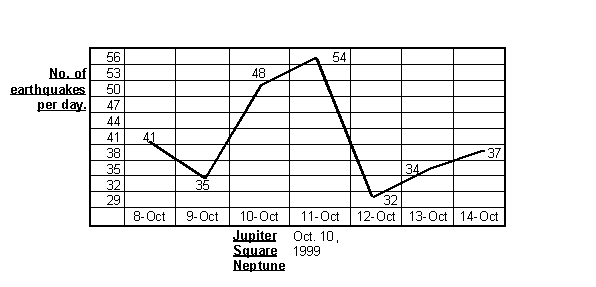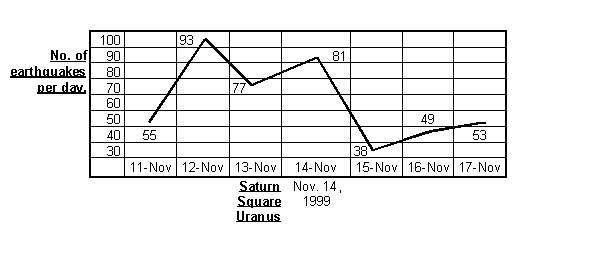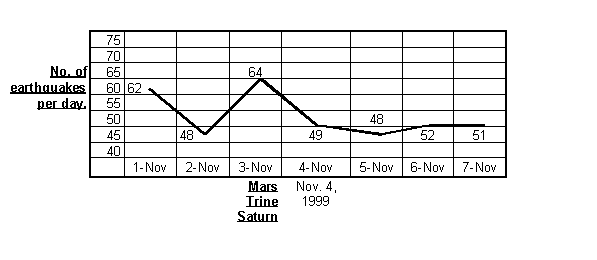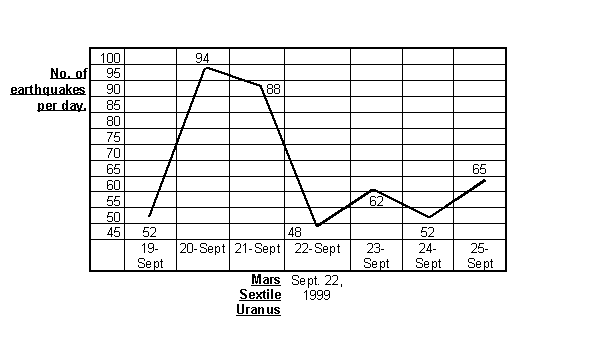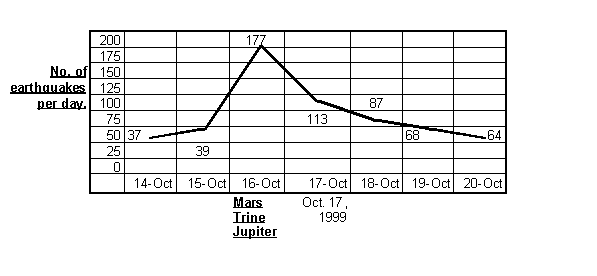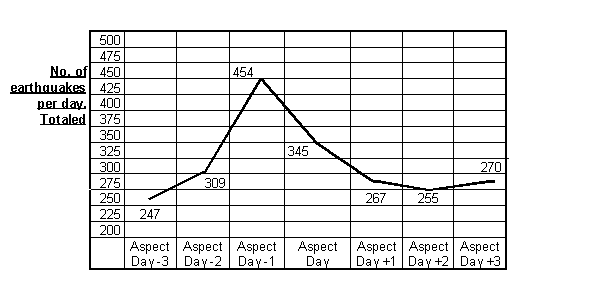The above series of graphs show the relative number of earthquakes that occurred three days prior to the given aspects, the day of the aspect and the three days following the aspect. The last graph is the sum of all of the planetary aspect graphs. The criteria for selecting the dates for the graphs and the aspects involved were as follows: a.) Only superior planets were used for the study, due to the fact that they represent the rarest type of aspect. This helped to isolate the influence of the aspect on the overall level of geo-seismicity, due to the relatively long duration of these events. b.) Pluto was not included due to its small size and enormous distance. c.) All aspects had to occur between September 1st, 1999 and December 1st, 1999.

The first graph shows the relative number of earthquakes per day during the period three days before the aspect Jupiter square Neptune, the day of the aspect, October 10th, 1999, and three days after the aspect. The peak of 54 earthquakes per day is actually one day after the actual aspect day. The graph does however, show a definite rise during the period around the aspect. The second graph shows the earthquake response to the aspect Saturn square Uranus. The peak in the number of earthquakes occurs on November 12th with 93 earthquakes on that day. The actual aspect occurred on November 14th. On that day the second highest peak occurred with 77 events. On the 15th of November the seismic activity dramatically dropped off and there were only 38 earthquakes. The next graph illustrates the seismic response to Mars trine Saturn. There is only a moderate rise in earthquakes with 64 events on November 3rd, 49 on the day of the aspect November 4th and 48 the day after. The next graph demonstrates a much more dramatic response for the aspect Mars sextile Saturn which occurred on September 22, 1999. There were 52 earthquakes on September 19th. This number rose to 94 events on September 20th. September 21st saw 88 events. This number dramatically dropped off on the actual day of the aspect to only 48 events, but rose the day after the aspect to 68. The most stunning response illustrating the relationship between planetary aspects and earthquakes is seen in the next graph. This aspect was Mars trine Jupiter, which occurred on October 17th, 1999. On the 14th and 15th respectively the number of earthquakes on each day was 37 and 39. This number peaked rapidly on the 16th with 177 events. On the 17th, the day of the aspect, there was 113 events and on the day after there was still 87 earthquakes. The activity gradually dropped off on the 19th and 20th with 68 and 64 events each day respectively.

The final graph illustrates beautifully the geo-seismic response to the planetary aspects as a whole. For this graph all of the earthquakes that occurred on each of the respective days prior to, during and after each aspect was added together and the result were graphed. On aspect day 3 the sum of the number of events is 247. On day 2 the number of earthquakes rises to 309. Day 1 continued the increase to 454 earthquakes. This number starts to decline on the aspect day to 345. Aspect day +1 continues this decline with a sum of 267 events which continues with only 255 events on day +2. The last day under study has a sum of 270 events. This graph clearly shows the relationship that exists between the aspects and earthquakes. It also illustrates that the peak actually occurs slightly before the time of the aspect, which confirms the earlier studies, that correlated applying aspects and increases in seismic activity.

When all the experiments and tests that have been presented up to this point in this paper are considered then there is virtually no chance that there is not a relationship between the planetary aspects and the level of siesmicity that the earth experiences. The results can be easily replicated, or disproved by any other researcher. Through all of the analysis that has been carried out upon this data there has been an extremely coherent internal consistency that maintains its integrity no matter how the data is sorted. The simple facts are that the larger the groups of earthquakes under study the greater the probability that 50% more earthquakes will occur before a planetary aspect happens than after the aspects reaches perfection.

Also that the peak in the rate of earthquake frequency will occur just before, or at the moment that the aspect actually occurs. There are more earthquakes that occur during periods of planetary aspects when compared to periods when there were none.

There are some rather unusual findings associated with this research that seem to contradict some suppositions that astrologers have had about aspects. For instance, none of the aspects seem any different than any of the other ones. All produce earthquakes. Other studies have indicated that all of the aspects of the 24th harmonic are about equal in producing earthquakes. This is in contradiction to the notion that "hard" aspects, the opposition, square, and conjunction should produce more earthquakes than "soft" aspects, such as the sextile and trine. The last study also indicated that time; as opposed to geometrical arc may be the relevant factor in determining when aspects are in effect. All of the aspects under study produced earthquakes for about the same period of time before the actual aspect time regardless of the relative motions of the planets. Traditional astrological theory states that slower moving planets, such as Saturn and Uranus should have longer lasting aspects than faster moving one, such as Mars and Jupiter. However Saturn square Uranus produced a similar curve in relation to aspects as did Mars trine Jupiter.

Other studies, which are beyond the scope of this present study, have shown that many other astrological factors such as the zodiac and whole sky patterns of planets have a strong effect upon when and where an earthquake will occur. However, no effect has been found with any of the asteroids, or the moons nodes. The rule of applying and separating aspects does not relate to any of the local space points, such as the ascendant, mid-heaven, part of fortune, vertex, or east point. These points may play a role in the exact positioning of the earthquake, not in relation to aspects, but rather in relation to zodiacal longitude.

The fact that planetary aspects do have an influence over the seismic activity of the earth is a major conundrum for conventional physics. The whole reason that physical science as has rejected astrology as a serious discipline is that Newtonian physics rules out effect at a distance. We must then devise a new theory as to how these events are being promulgated. It would seem that gravitation is the only logical way that there could be an influence by the planets upon the earth. The force that the planets exert upon the earth is so minute that gravitation alone cannot be responsible for the observed effect. There must then be a harmonic effect that magnifies the gravitational effects of the planets upon the earth. The time space matrix is distorted by the presence of matter within it. For us here in the Milky Way the primary distortion around us is produced by the nucleus of the galaxy, which contains most of the galaxies mass. In local space the gravitational fields of the stars around us distort gravity. This in turn sets up standing gravitational wave forms through which the planets and the solar system as a whole traverse. These standing waves must allow a gravitational amplification of force that acts upon the earth as the planets form perfect angles with it. It may be that the planets act like transistors, with the emitter being the extrasolar systemic gravitational matrix, the base being the planetary aspect and the collector being the earth. Possibly it could be viewed another way. The emitter could be the outermost planet, the base could be the intermediary planet and the collector could be the earth. The aspect in that case would be the bias that would allow the transmission of gravitational force through the standing waves of the cosmic gravitational field. This type of gravitational harmonic action is seen in many instances throughout the solar system. Some examples being the harmonic spacing of the asteroids with the orbit of Jupiter, the spacing of Jupiters moons orbits themselves and the spacing of the rings of Saturn and it many strange moons.

Regardless what the mechanism is behind the effects of the planetary aspects the result from the studies are clear: the planetary mutual aspects create an increase in geoseismic activity. A great deal more research must be carried out before the exact relationships and the underlying forces are fully understood.

Ed. N.: You can find more information about the data upon which the article is based by contacting Brian T. Johnston .